Скачать презентацию Automated Reasoning Systems For first order Predicate Logic

a0d8f5a20e308a72be172fa9c35844dc.ppt

• Количество слайдов: 49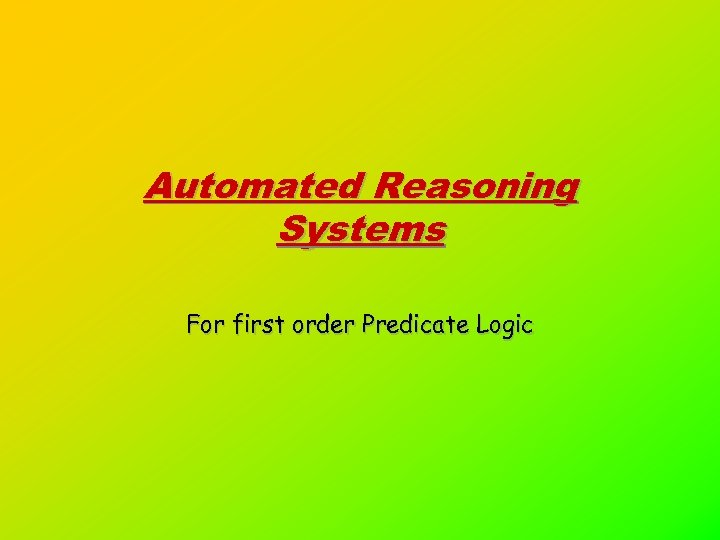Automated Reasoning Systems For first order Predicate Logic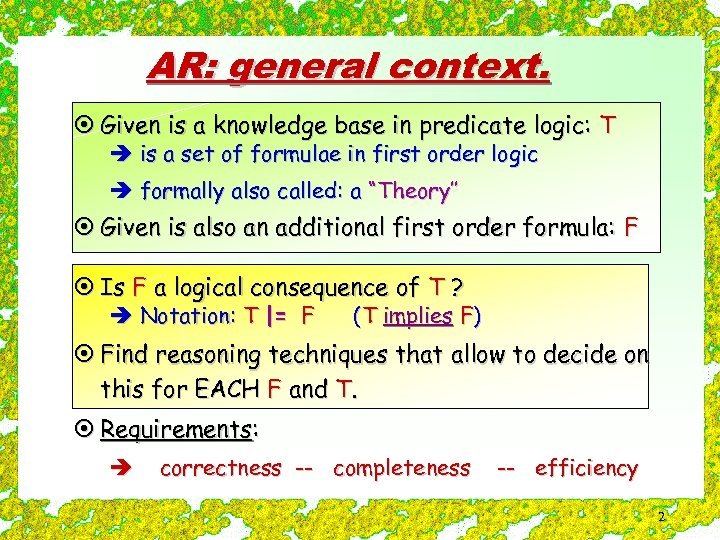AR: general context. ¤ Given is a knowledge base in predicate logic: T è is a set of formulae in first order logic è formally also called: a “Theory’’ ¤ Given is also an additional first order formula: F ¤ Is F a logical consequence of T ? è Notation: T |= F (T implies F) ¤ Find reasoning techniques that allow to decide on this for EACH F and T. ¤ Requirements: è correctness -- completeness -- efficiency 2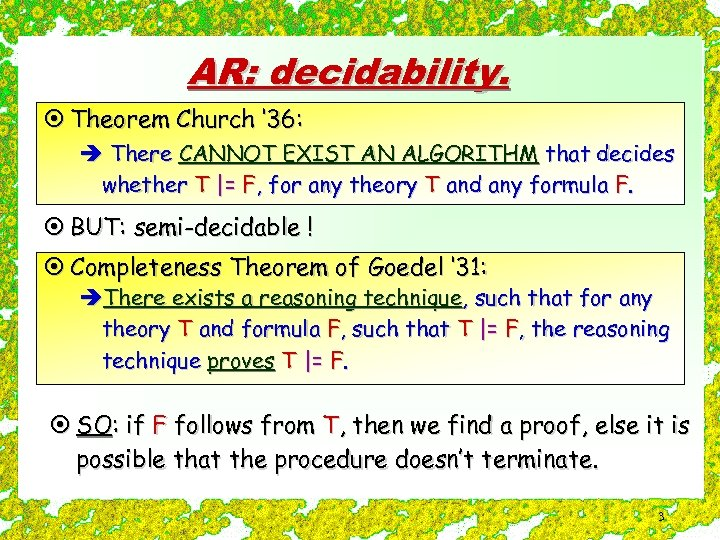AR: decidability. ¤ Theorem Church ‘ 36: è There CANNOT EXIST AN ALGORITHM that decides whether T |= F, for any theory T and any formula F. ¤ BUT: semi-decidable ! ¤ Completeness Theorem of Goedel ‘ 31: èThere exists a reasoning technique, such that for any theory T and formula F, such that T |= F, the reasoning technique proves T |= F. ¤ SO: if F follows from T, then we find a proof, else it is possible that the procedure doesn’t terminate. 3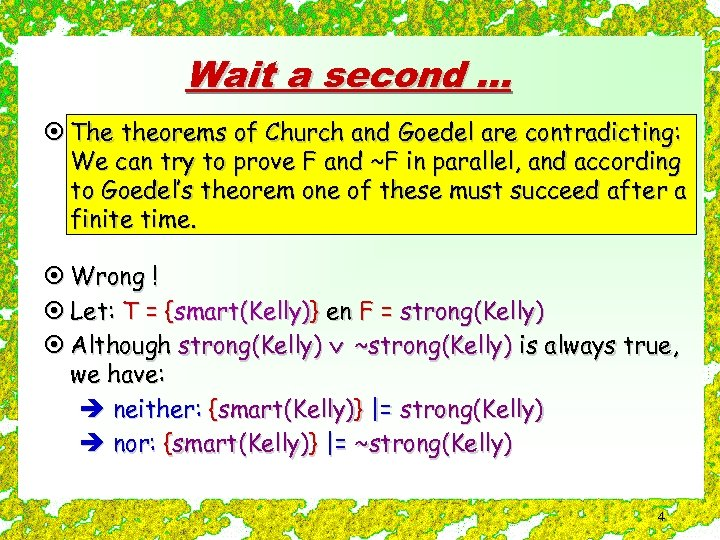Wait a second. . . ¤ The theorems of Church and Goedel are contradicting: We can try to prove F and ~F in parallel, and according to Goedel’s theorem one of these must succeed after a finite time. ¤ Wrong ! ¤ Let: T = {smart(Kelly)} en F = strong(Kelly) ¤ Although strong(Kelly) ~strong(Kelly) is always true, we have: è neither: {smart(Kelly)} |= strong(Kelly) è nor: {smart(Kelly)} |= ~strong(Kelly) 4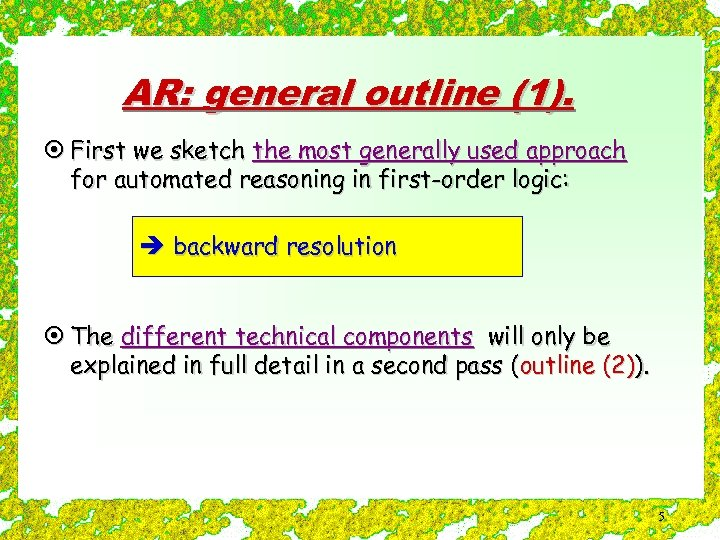AR: general outline (1). ¤ First we sketch the most generally used approach for automated reasoning in first-order logic: è backward resolution ¤ The different technical components will only be explained in full detail in a second pass (outline (2)). 5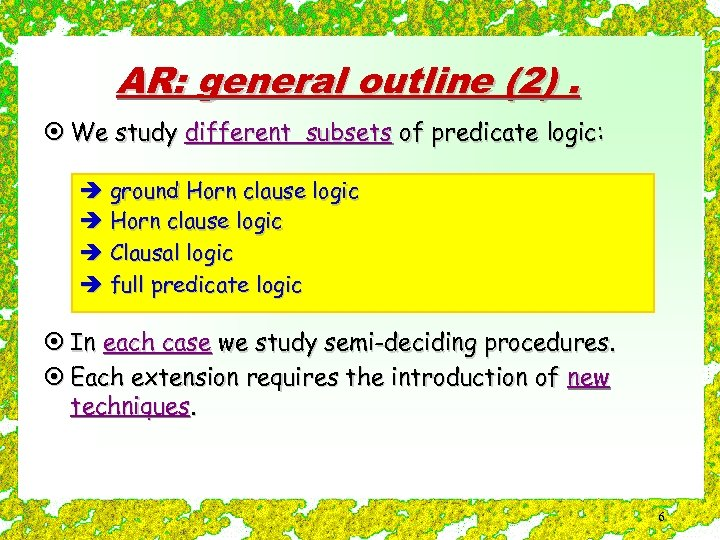AR: general outline (2). ¤ We study different subsets of predicate logic: è ground Horn clause logic è Clausal logic è full predicate logic ¤ In each case we study semi-deciding procedures. ¤ Each extension requires the introduction of new techniques. 6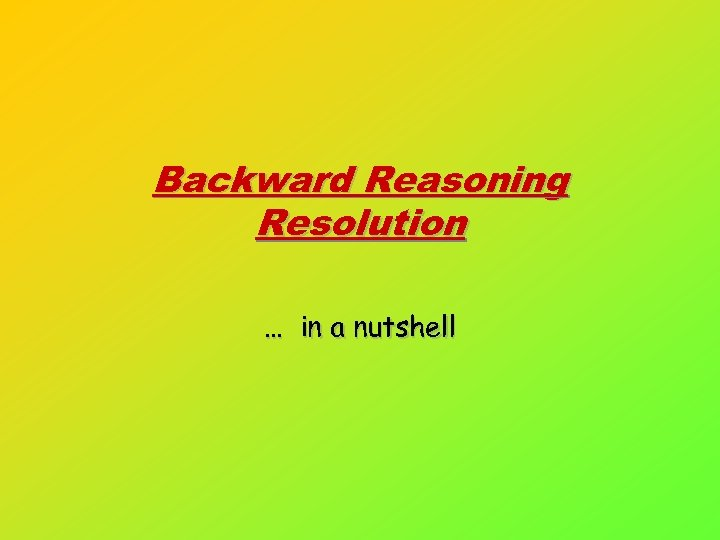Backward Reasoning Resolution … in a nutshell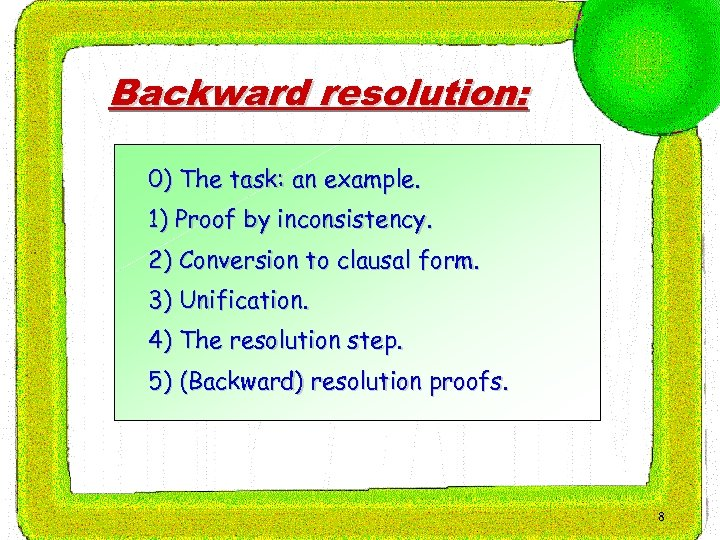Backward resolution: 0) The task: an example. 1) Proof by inconsistency. 2) Conversion to clausal form. 3) Unification. 4) The resolution step. 5) (Backward) resolution proofs. 8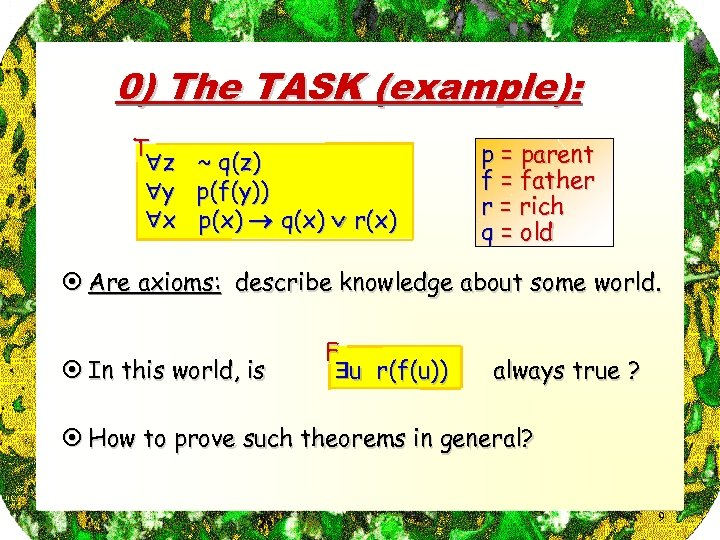0) The TASK (example): T z y x ~ q(z) p(f(y)) p(x) q(x) r(x) p = parent f = father r = rich q = old ¤ Are axioms: describe knowledge about some world. ¤ In this world, is F u r(f(u)) always true ? ¤ How to prove such theorems in general? 9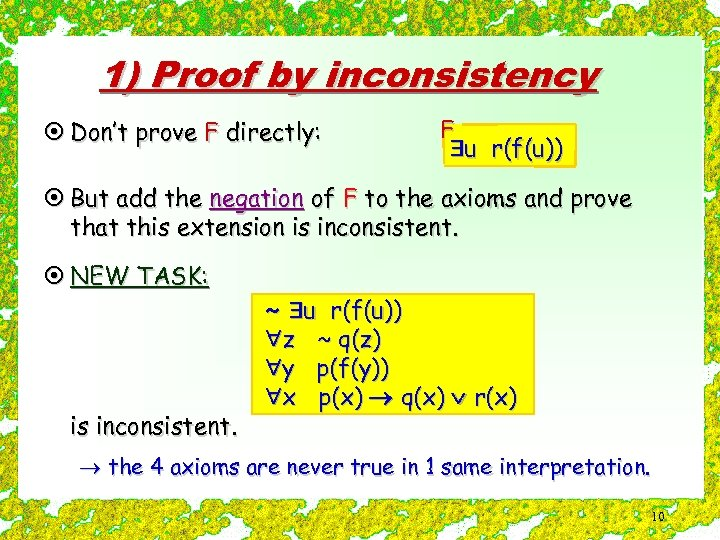1) Proof by inconsistency ¤ Don’t prove F directly: F u r(f(u)) ¤ But add the negation of F to the axioms and prove that this extension is inconsistent. ¤ NEW TASK: is inconsistent. ~ u r(f(u)) z ~ q(z) y p(f(y)) x p(x) q(x) r(x) ® the 4 axioms are never true in 1 same interpretation. 10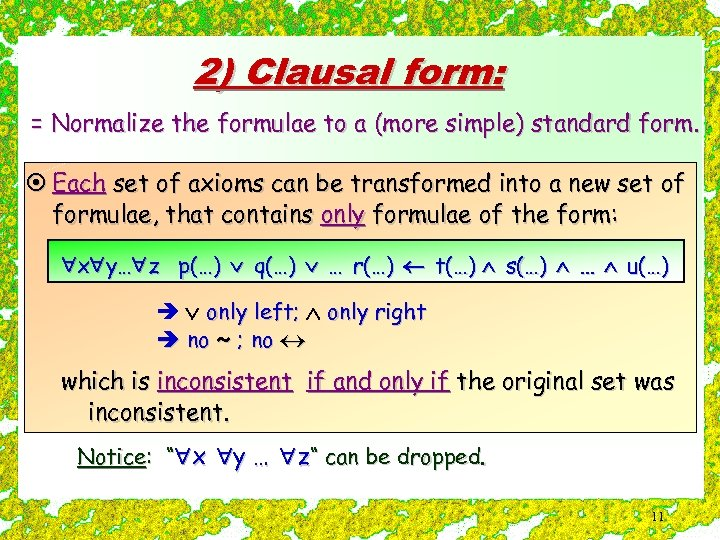2) Clausal form: = Normalize the formulae to a (more simple) standard form. ¤ Each set of axioms can be transformed into a new set of formulae, that contains only formulae of the form: x y… z p(…) q(…) … r(…) t(…) s(…) … u(…) è only left; only right è no ~ ; no which is inconsistent if and only if the original set was inconsistent. Notice: “ x y … z“ can be dropped. 11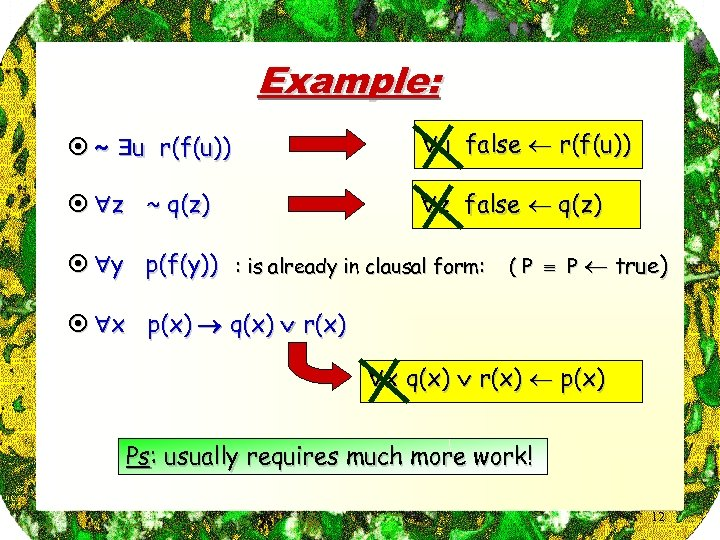Example: ¤ ~ u r(f(u)) u false r(f(u)) ¤ z ~ q(z) z false q(z) ¤ y p(f(y)) : is already in clausal form: ( P P true) ¤ x p(x) q(x) r(x) x q(x) r(x) p(x) Ps: usually requires much more work! 12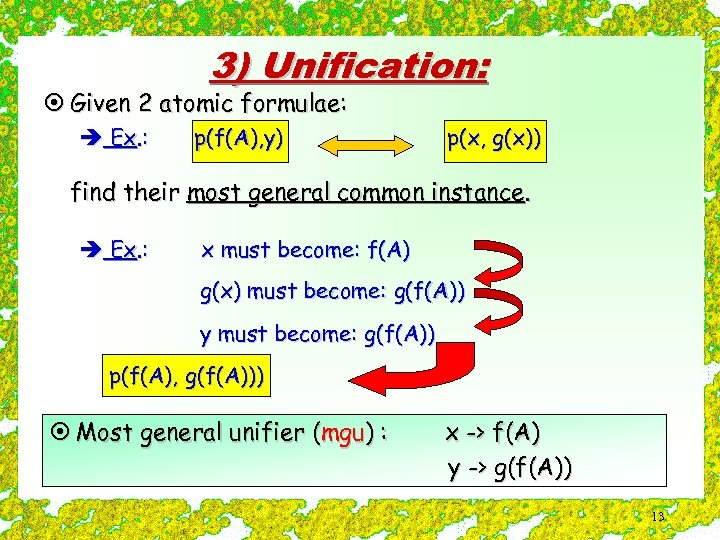3) Unification: ¤ Given 2 atomic formulae: è Ex. : p(f(A), y) p(x, g(x)) find their most general common instance. è Ex. : x must become: f(A) g(x) must become: g(f(A)) y must become: g(f(A)) p(f(A), g(f(A))) ¤ Most general unifier (mgu) : x -> f(A) y -> g(f(A)) 13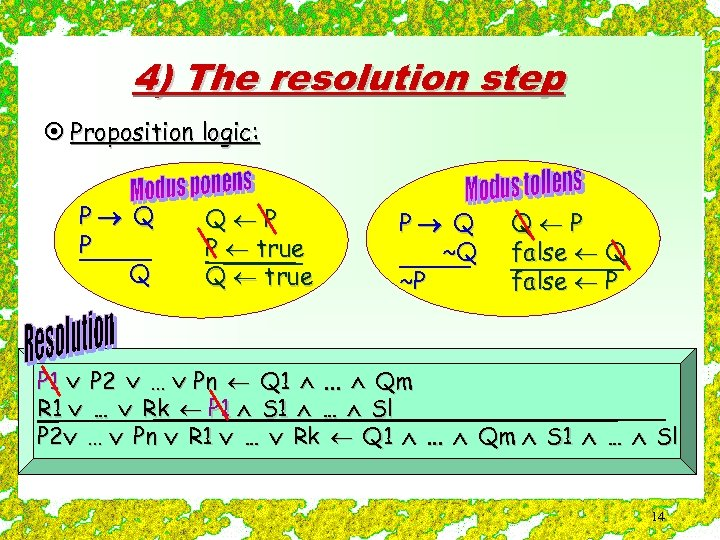4) The resolution step ¤ Proposition logic: P Q Q P P true Q true P Q ~Q ~P Q P false Q false P P 1 P 2 … Pn Q 1 . . . Qm R 1 … Rk P 1 S 1 … Sl P 2 … Pn R 1 … Rk Q 1 . . . Qm S 1 … Sl 14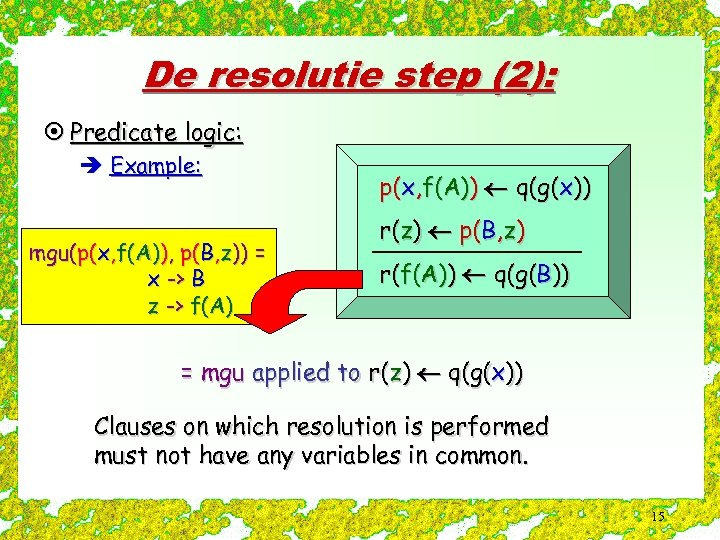De resolutie step (2): ¤ Predicate logic: è Example: mgu(p(x, f(A)), p(B, z)) = x -> B z -> f(A) p(x, f(A)) q(g(x)) r(z) p(B, z) r(f(A)) q(g(B)) = mgu applied to r(z) q(g(x)) Clauses on which resolution is performed must not have any variables in common. 15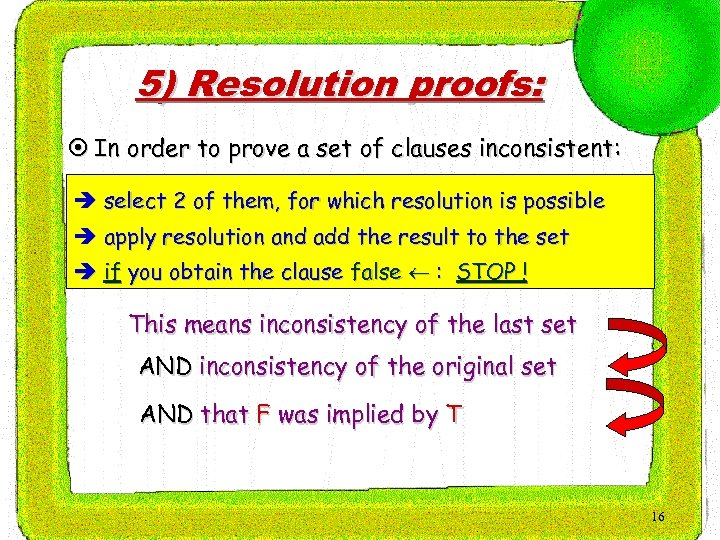5) Resolution proofs: ¤ In order to prove a set of clauses inconsistent: è select 2 of them, for which resolution is possible è apply resolution and add the result to the set è if you obtain the clause false : STOP ! This means inconsistency of the last set AND inconsistency of the original set AND that F was implied by T 16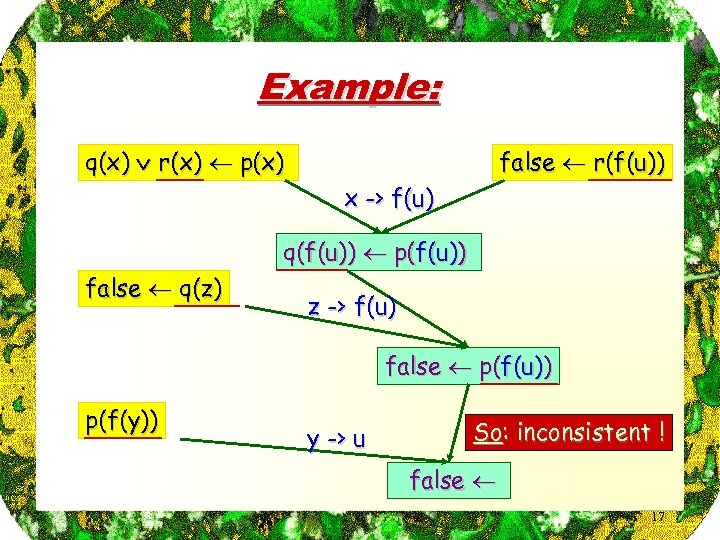Example: q(x) r(x) p(x) false r(f(u)) x -> f(u) q(f(u)) p(f(u)) false q(z) z -> f(u) false p(f(u)) p(f(y)) y -> u So: inconsistent ! false 17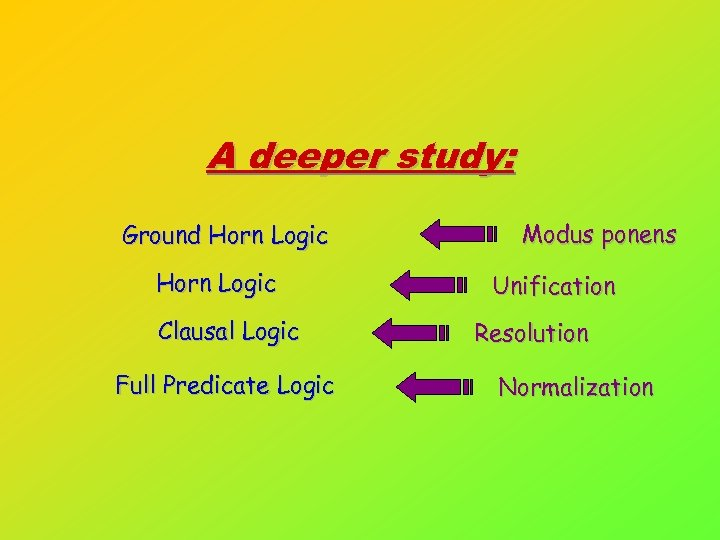A deeper study: Ground Horn Logic Clausal Logic Full Predicate Logic Modus ponens Unification Resolution Normalization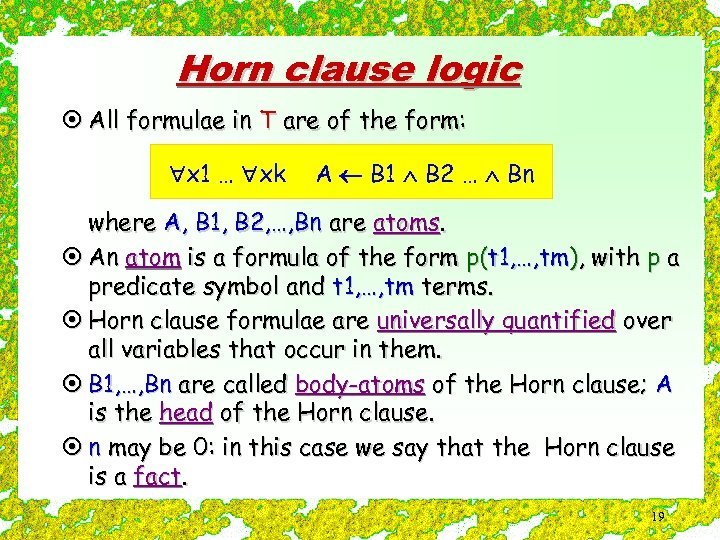Horn clause logic ¤ All formulae in T are of the form: x 1 … xk A B 1 B 2 … Bn where A, B 1, B 2, …, Bn are atoms. ¤ An atom is a formula of the form p(t 1, …, tm), with p a predicate symbol and t 1, …, tm terms. ¤ Horn clause formulae are universally quantified over all variables that occur in them. ¤ B 1, …, Bn are called body-atoms of the Horn clause; A is the head of the Horn clause. ¤ n may be 0: in this case we say that the Horn clause is a fact. 19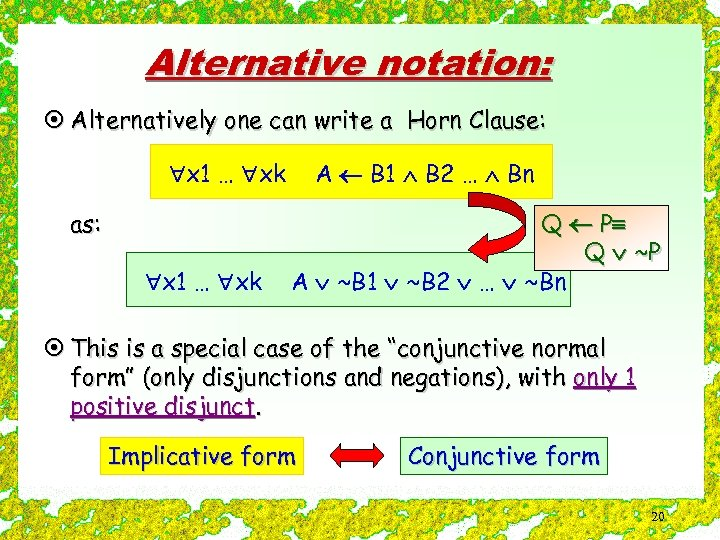Alternative notation: ¤ Alternatively one can write a Horn Clause: x 1 … xk as: x 1 … xk A B 1 B 2 … Bn Q P Q ~P A ~B 1 ~B 2 … ~Bn ¤ This is a special case of the “conjunctive normal form” (only disjunctions and negations), with only 1 positive disjunct. Implicative form Conjunctive form 20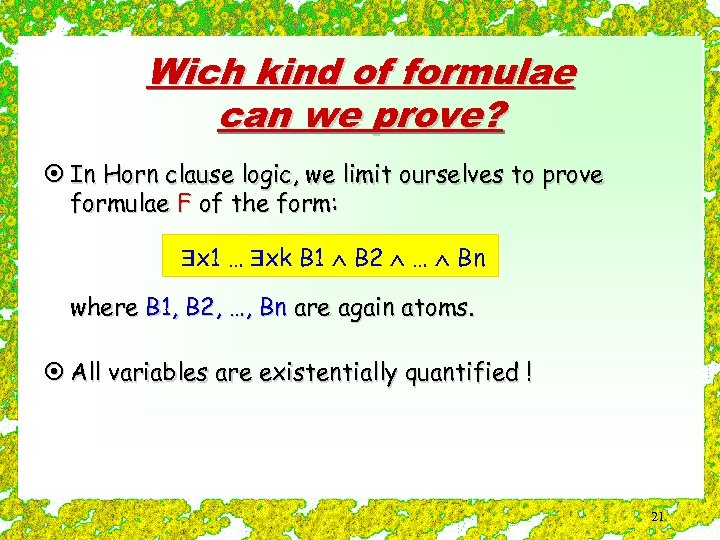Wich kind of formulae can we prove? ¤ In Horn clause logic, we limit ourselves to prove formulae F of the form: x 1 … xk B 1 B 2 … Bn where B 1, B 2, …, Bn are again atoms. ¤ All variables are existentially quantified ! 21A very simple example: ¤ Bosmans is a showmaster (1) ¤ Showmasters are rich (2) ¤ Rich people have big houses (3) ¤ Big houses need a lot of maintenance (4) èGoal: automatically deduce that Bosmans’ house needs a lot of maintenance. 22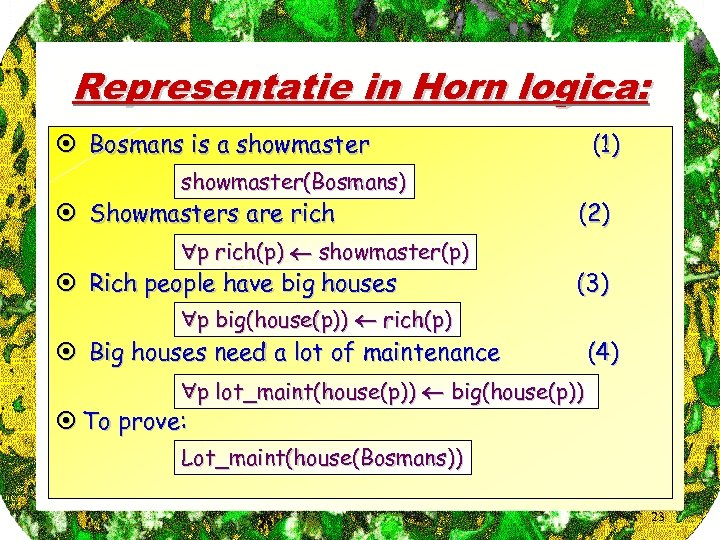Representatie in Horn logica: ¤ Bosmans is a showmaster(Bosmans) ¤ Showmasters are rich p rich(p) showmaster(p) ¤ Rich people have big houses (1) (2) (3) p big(house(p)) rich(p) ¤ Big houses need a lot of maintenance (4) p lot_maint(house(p)) big(house(p)) ¤ To prove: Lot_maint(house(Bosmans)) 23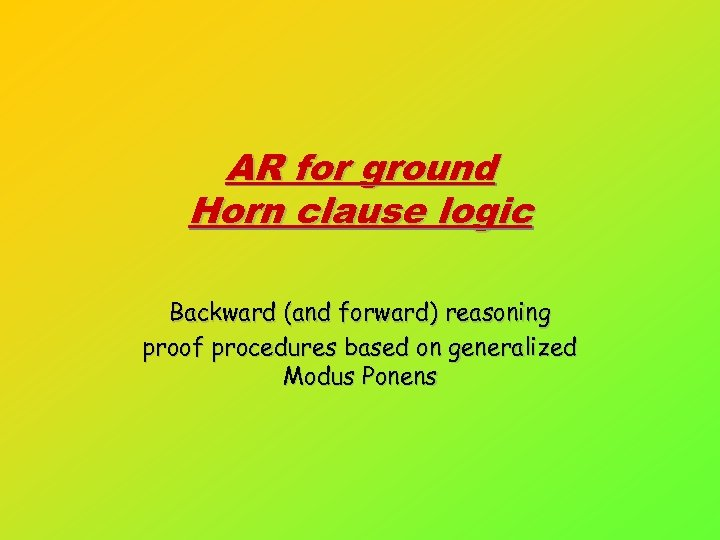AR for ground Horn clause logic Backward (and forward) reasoning proof procedures based on generalized Modus Ponens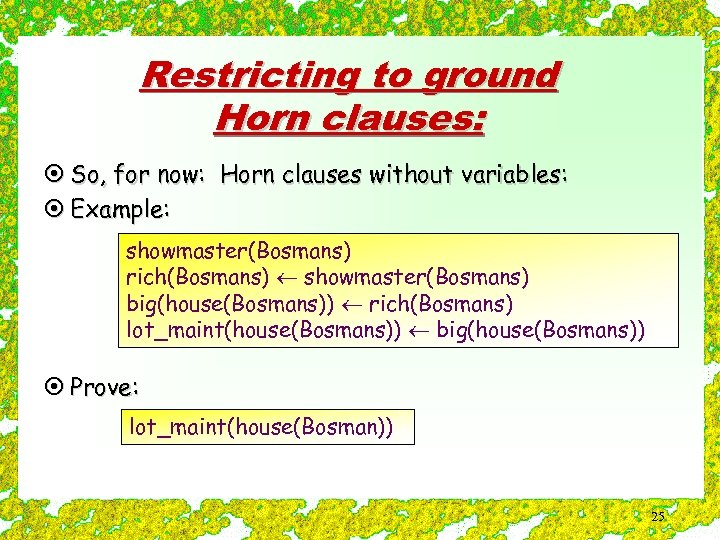Restricting to ground Horn clauses: ¤ So, for now: Horn clauses without variables: ¤ Example: showmaster(Bosmans) rich(Bosmans) showmaster(Bosmans) big(house(Bosmans)) rich(Bosmans) lot_maint(house(Bosmans)) big(house(Bosmans)) ¤ Prove: lot_maint(house(Bosman)) 25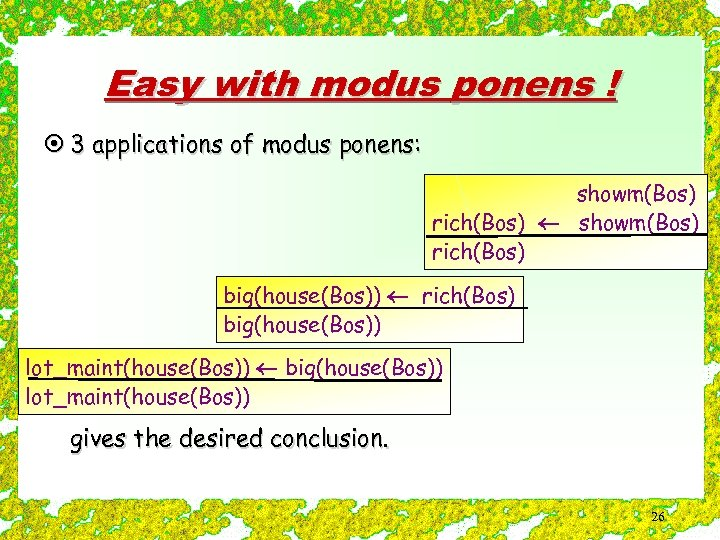Easy with modus ponens ! ¤ 3 applications of modus ponens: showm(Bos) rich(Bos) big(house(Bos)) lot_maint(house(Bos)) gives the desired conclusion. 26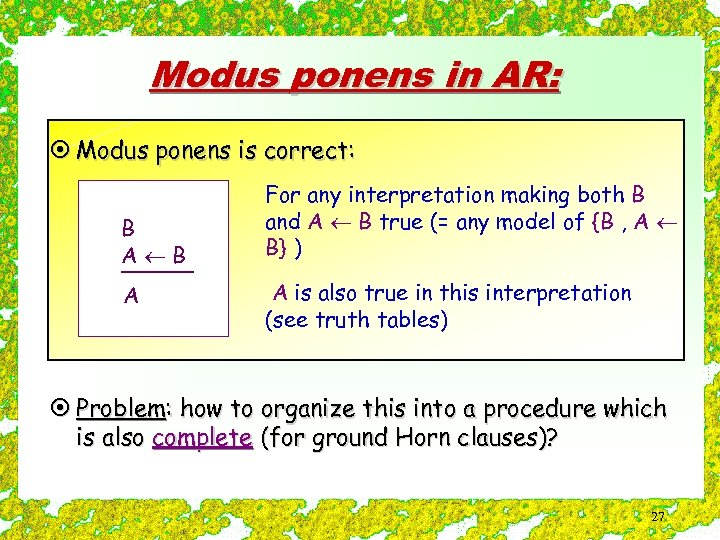Modus ponens in AR: ¤ Modus ponens is correct: B A For any interpretation making both B and A B true (= any model of {B , A B} ) A is also true in this interpretation (see truth tables) ¤ Problem: how to organize this into a procedure which is also complete (for ground Horn clauses)? 27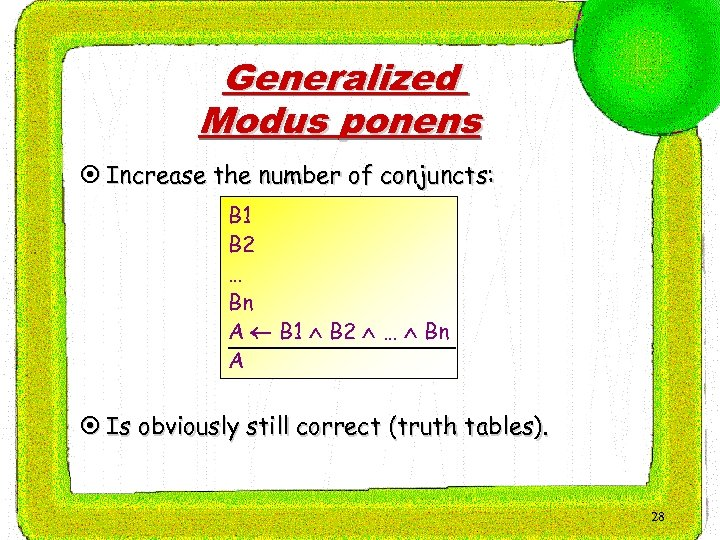Generalized Modus ponens ¤ Increase the number of conjuncts: B 1 B 2 … Bn A B 1 B 2 … Bn A ¤ Is obviously still correct (truth tables). 28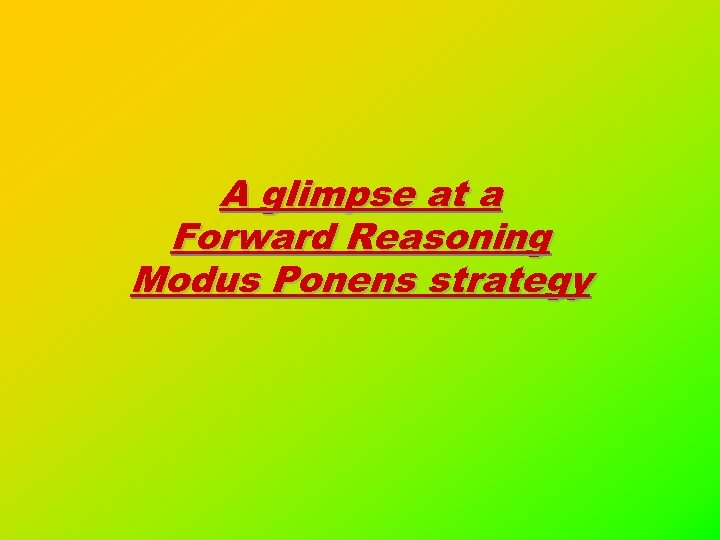A glimpse at a Forward Reasoning Modus Ponens strategy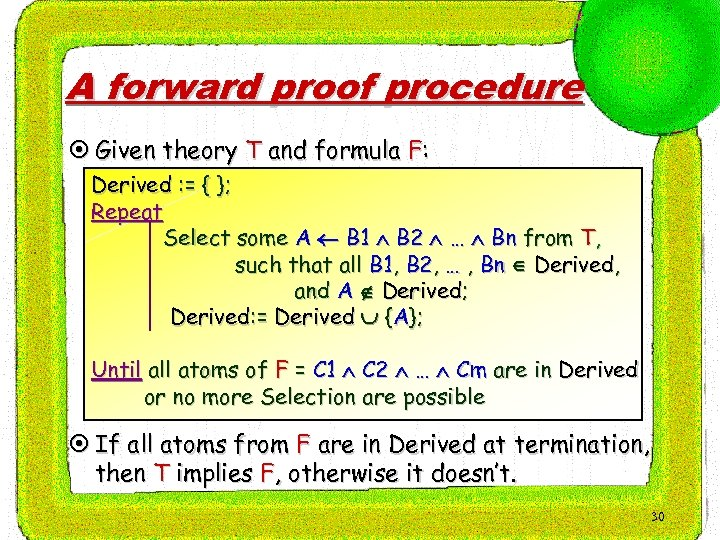A forward proof procedure ¤ Given theory T and formula F: Derived : = { }; Repeat Select some A B 1 B 2 … Bn from T, such that all B 1, B 2, … , Bn Derived, and A Derived; Derived: = Derived {A}; Until all atoms of F = C 1 C 2 … Cm are in Derived or no more Selection are possible ¤ If all atoms from F are in Derived at termination, then T implies F, otherwise it doesn’t. 30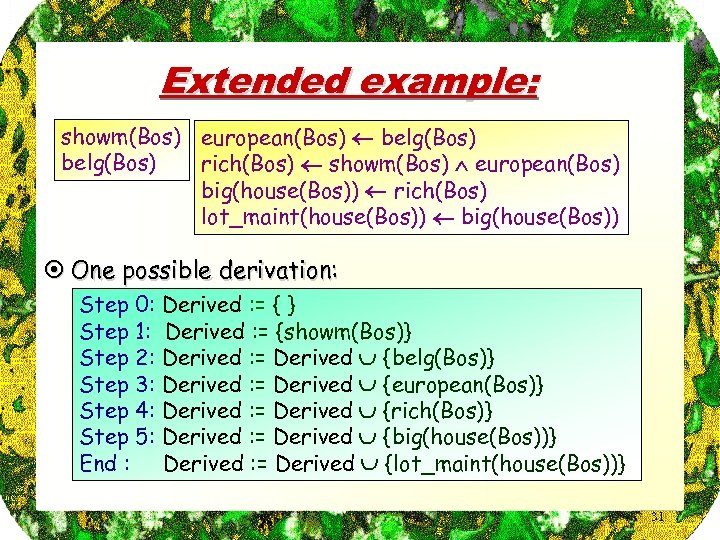Extended example: showm(Bos) european(Bos) belg(Bos) rich(Bos) showm(Bos) european(Bos) big(house(Bos)) rich(Bos) lot_maint(house(Bos)) big(house(Bos)) ¤ One possible derivation: Step 0: Step 1: Step 2: Step 3: Step 4: Step 5: End : Derived : = { } Derived : = {showm(Bos)} Derived : = Derived {belg(Bos)} Derived : = Derived {european(Bos)} Derived : = Derived {rich(Bos)} Derived : = Derived {big(house(Bos))} Derived : = Derived {lot_maint(house(Bos))} 31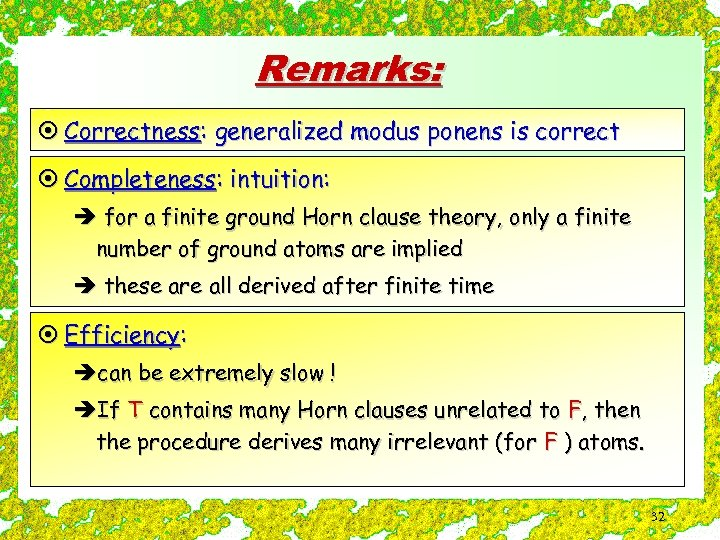Remarks: ¤ Correctness: generalized modus ponens is correct ¤ Completeness: intuition: è for a finite ground Horn clause theory, only a finite number of ground atoms are implied è these are all derived after finite time ¤ Efficiency: ècan be extremely slow ! èIf T contains many Horn clauses unrelated to F, then the procedure derives many irrelevant (for F ) atoms. 32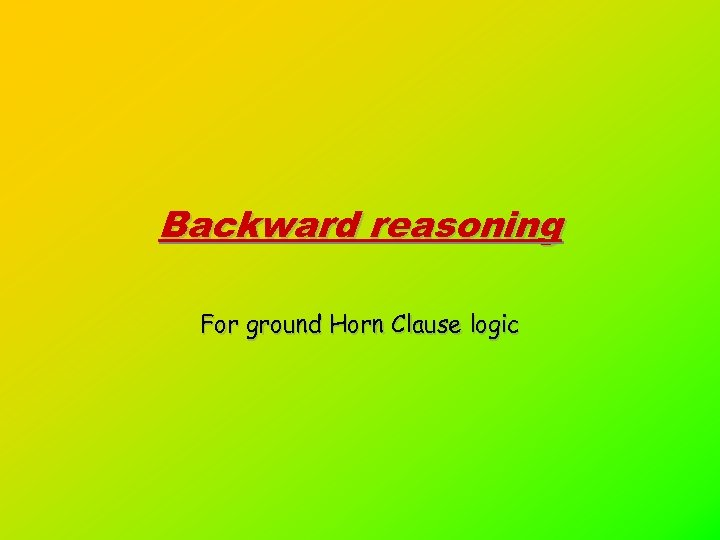Backward reasoning For ground Horn Clause logic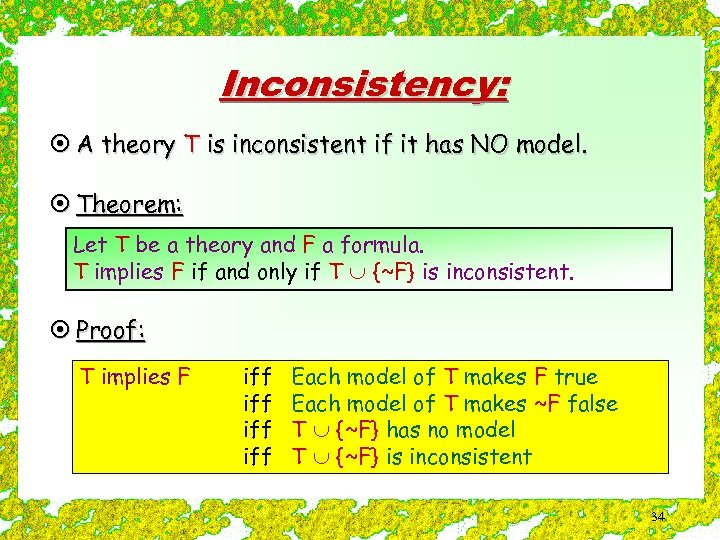Inconsistency: ¤ A theory T is inconsistent if it has NO model. ¤ Theorem: Let T be a theory and F a formula. T implies F if and only if T {~F} is inconsistent. ¤ Proof: T implies F iff iff Each model of T makes F true Each model of T makes ~F false T {~F} has no model T {~F} is inconsistent 34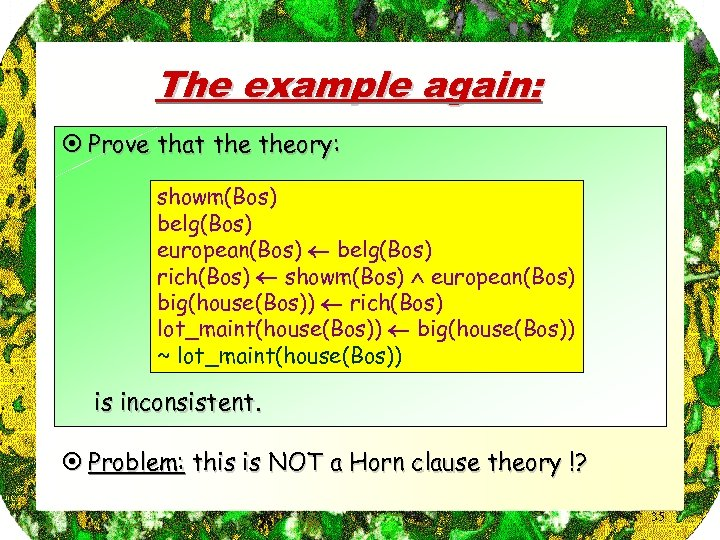The example again: ¤ Prove that theory: showm(Bos) belg(Bos) european(Bos) belg(Bos) rich(Bos) showm(Bos) european(Bos) big(house(Bos)) rich(Bos) lot_maint(house(Bos)) big(house(Bos)) ~ lot_maint(house(Bos)) is inconsistent. ¤ Problem: this is NOT a Horn clause theory !? 35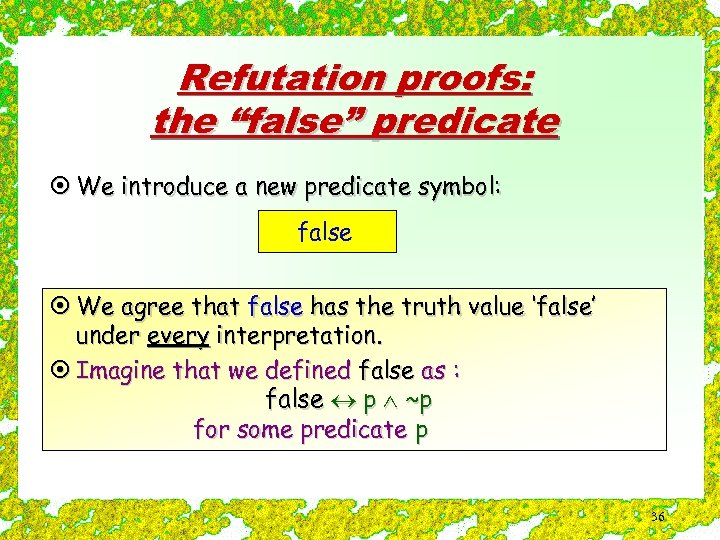Refutation proofs: the “false” predicate ¤ We introduce a new predicate symbol: false ¤ We agree that false has the truth value ‘false’ under every interpretation. ¤ Imagine that we defined false as : false p ~p for some predicate p 36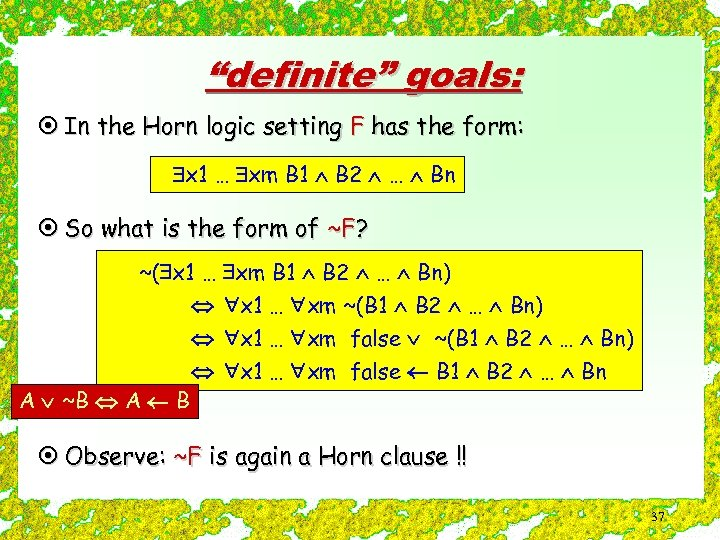“definite” goals: ¤ In the Horn logic setting F has the form: x 1 … xm B 1 B 2 … Bn ¤ So what is the form of ~F? ~( x 1 … xm B 1 B 2 … Bn) x 1 … xm ~(B 1 B 2 … Bn) A ~B A B x 1 … xm false ~(B 1 B 2 … Bn) x 1 … xm false B 1 B 2 … Bn ¤ Observe: ~F is again a Horn clause !! 37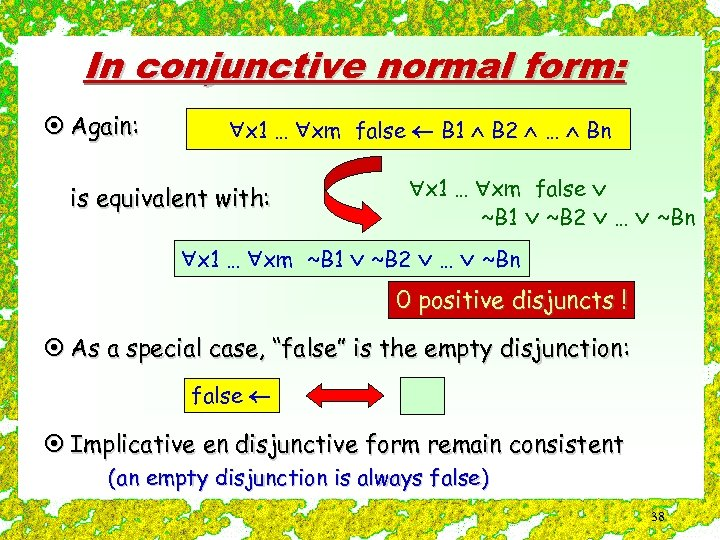In conjunctive normal form: ¤ Again: x 1 … xm false B 1 B 2 … Bn is equivalent with: x 1 … xm false ~B 1 ~B 2 … ~Bn x 1 … xm ~B 1 ~B 2 … ~Bn 0 positive disjuncts ! ¤ As a special case, “false” is the empty disjunction: false ¤ Implicative en disjunctive form remain consistent (an empty disjunction is always false) 38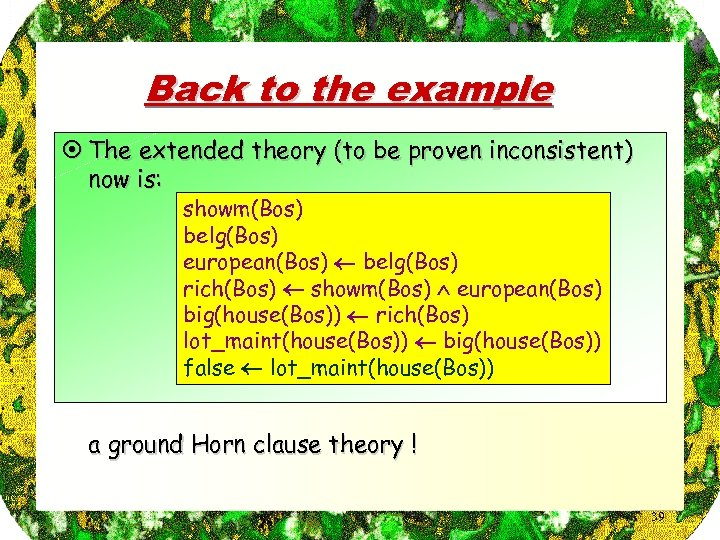Back to the example ¤ The extended theory (to be proven inconsistent) now is: showm(Bos) belg(Bos) european(Bos) belg(Bos) rich(Bos) showm(Bos) european(Bos) big(house(Bos)) rich(Bos) lot_maint(house(Bos)) big(house(Bos)) false lot_maint(house(Bos)) a ground Horn clause theory ! 39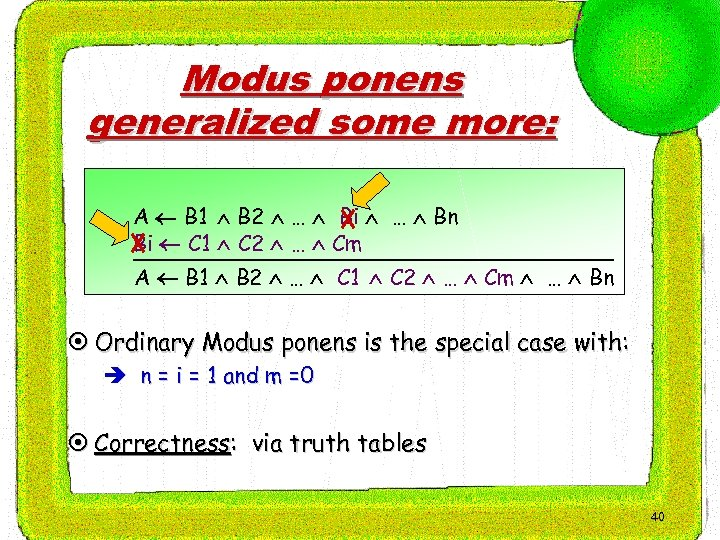Modus ponens generalized some more: A B 1 B 2 … Bi … Bn Bi C 1 C 2 … Cm A B 1 B 2 … C 1 C 2 … Cm … Bn ¤ Ordinary Modus ponens is the special case with: è n = i = 1 and m =0 ¤ Correctness: via truth tables 40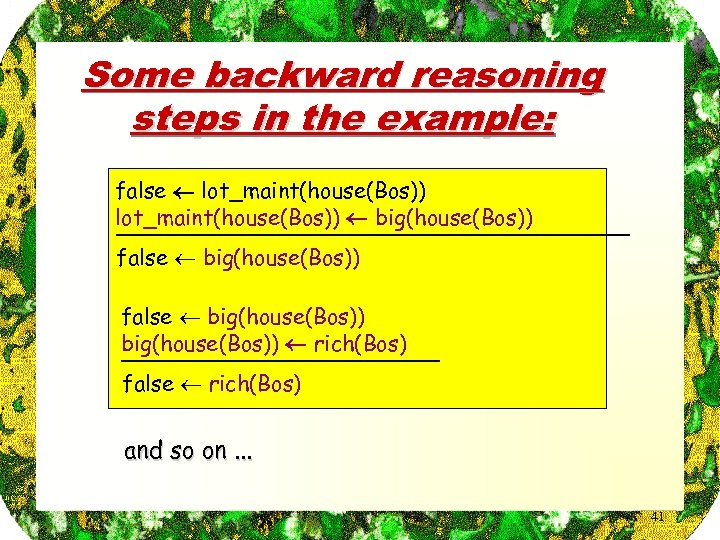Some backward reasoning steps in the example: false lot_maint(house(Bos)) big(house(Bos)) false big(house(Bos)) rich(Bos) false rich(Bos) and so on. . . 41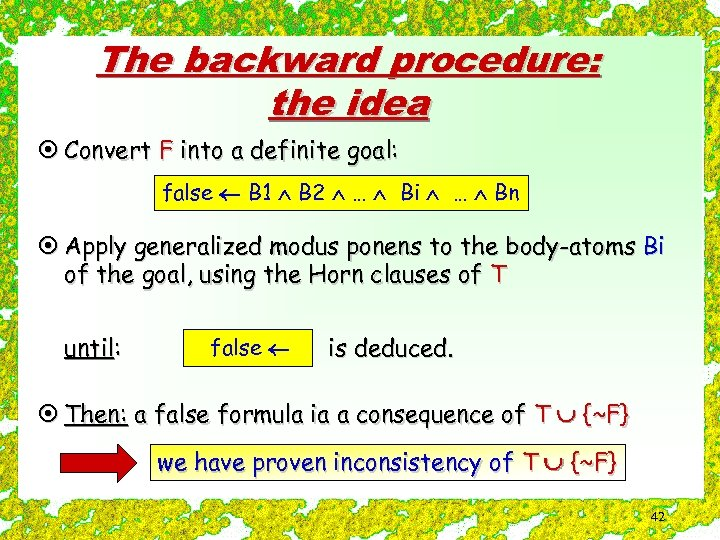The backward procedure: the idea ¤ Convert F into a definite goal: false B 1 B 2 … Bi … Bn ¤ Apply generalized modus ponens to the body-atoms Bi of the goal, using the Horn clauses of T until: false is deduced. ¤ Then: a false formula ia a consequence of T {~F} we have proven inconsistency of T {~F} 42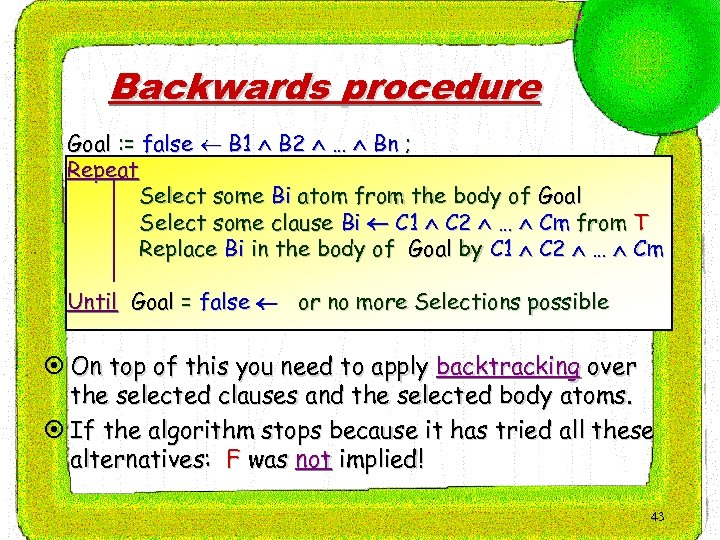Backwards procedure Goal : = false B 1 B 2 … Bn ; Repeat Select some Bi atom from the body of Goal Select some clause Bi C 1 C 2 … Cm from T Replace Bi in the body of Goal by C 1 C 2 … Cm Until Goal = false or no more Selections possible ¤ On top of this you need to apply backtracking over the selected clauses and the selected body atoms. ¤ If the algorithm stops because it has tried all these alternatives: F was not implied! 43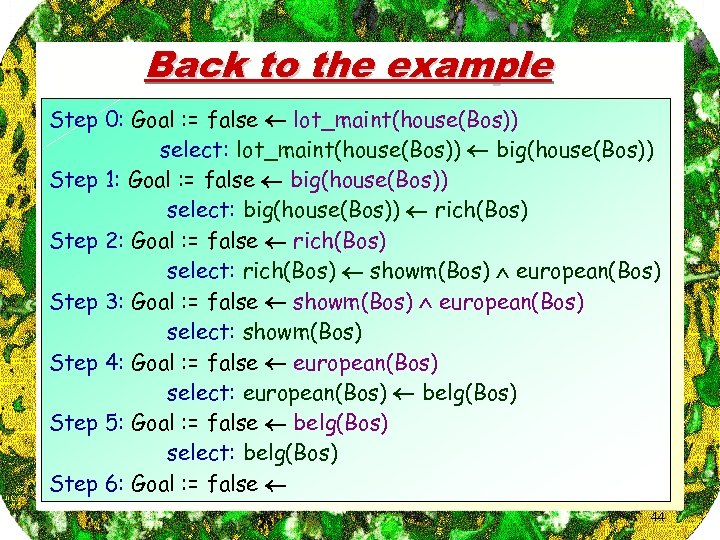Back to the example Step 0: Goal : = false lot_maint(house(Bos)) select: lot_maint(house(Bos)) big(house(Bos)) Step 1: Goal : = false big(house(Bos)) select: big(house(Bos)) rich(Bos) Step 2: Goal : = false rich(Bos) select: rich(Bos) showm(Bos) european(Bos) Step 3: Goal : = false showm(Bos) european(Bos) select: showm(Bos) Step 4: Goal : = false european(Bos) select: european(Bos) belg(Bos) Step 5: Goal : = false belg(Bos) select: belg(Bos) Step 6: Goal : = false 44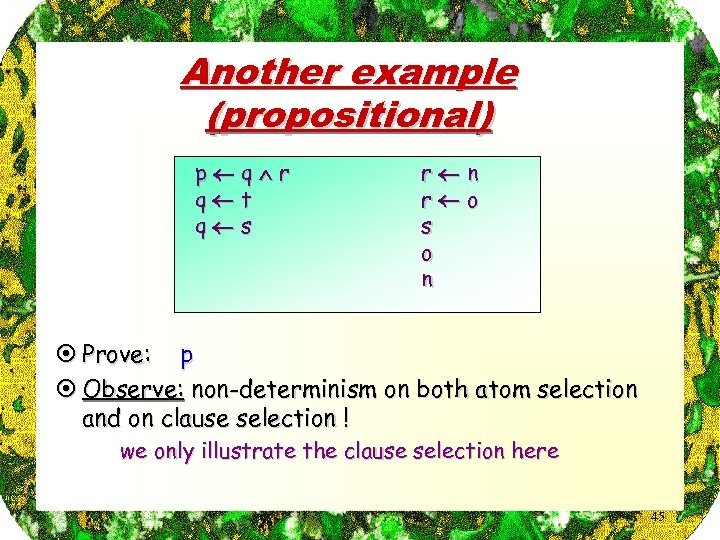Another example (propositional) p q r q t q s r n r o s o n ¤ Prove: p ¤ Observe: non-determinism on both atom selection and on clause selection ! we only illustrate the clause selection here 45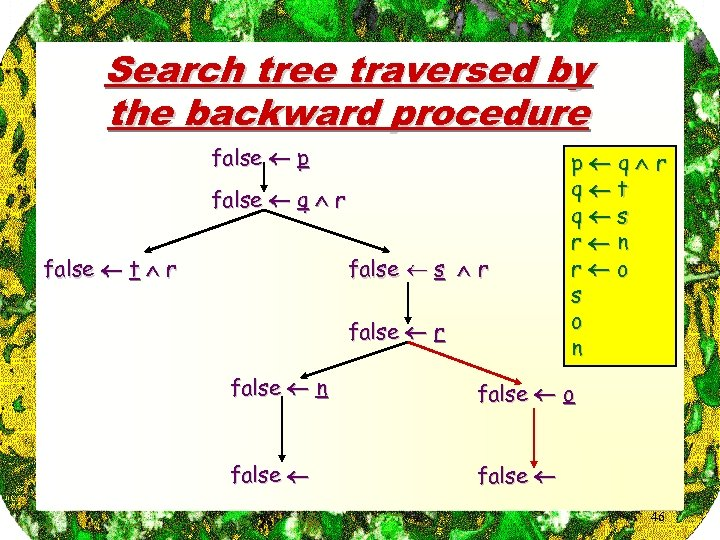Search tree traversed by the backward procedure false p false q r false t r false s r false r p q r q t q s r n r o s o n false o false 46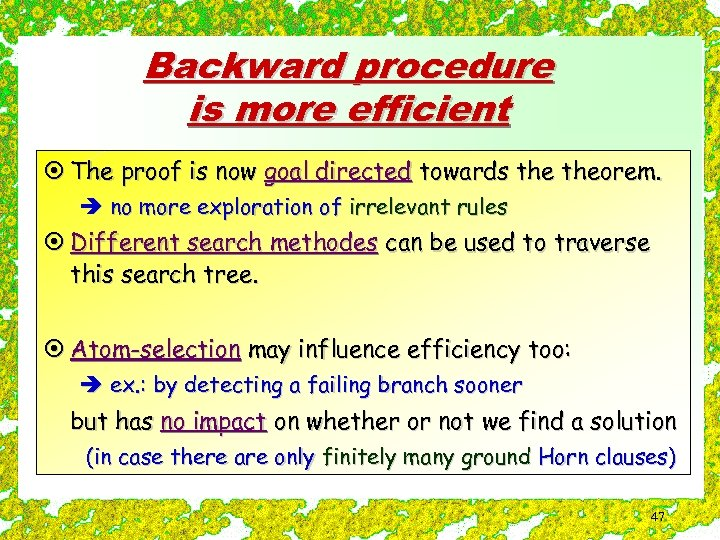Backward procedure is more efficient ¤ The proof is now goal directed towards theorem. è no more exploration of irrelevant rules ¤ Different search methodes can be used to traverse this search tree. ¤ Atom-selection may influence efficiency too: è ex. : by detecting a failing branch sooner but has no impact on whether or not we find a solution (in case there are only finitely many ground Horn clauses) 47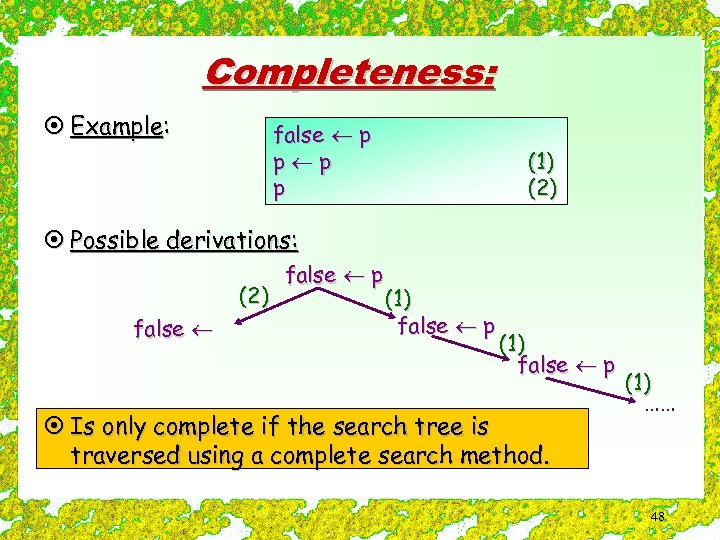Completeness: ¤ Example: false p p (1) (2) ¤ Possible derivations: (2) false p (1) false p ¤ Is only complete if the search tree is traversed using a complete search method. (1) …… 48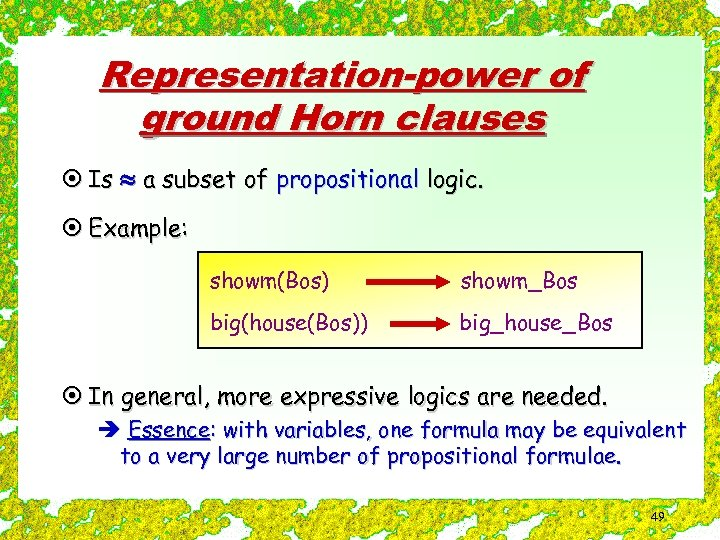Representation-power of ground Horn clauses ¤ Is a subset of propositional logic. ¤ Example: showm(Bos) showm_Bos big(house(Bos)) big_house_Bos ¤ In general, more expressive logics are needed. è Essence: with variables, one formula may be equivalent to a very large number of propositional formulae. 49